### IMO Shortlist 2005 problem N7

Kvaliteta:
Avg: 3.0
Težina:
Avg: 9.0
Let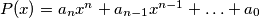$P(x)=a_{n}x^{n}+a_{n-1}x^{n-1}+\ldots+a_{0}$, where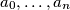$a_{0},\ldots,a_{n}$ are integers,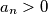$a_{n}>0$,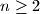$n\geq 2$. Prove that there exists a positive integer$m$ such that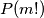$P(m!)$ is a composite number.
Source: Međunarodna matematička olimpijada, shortlist 2005